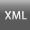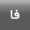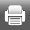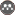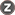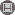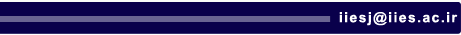[Home ] [Archive]   [ فارسی ]Volume 15, Issue 63 (Winter 1398 2020)QEER 2020, 15(63): 243-267 Back to browse issues page
Development of Markov Chain Grey Regression Model to Forecast the Annual Natural Gas Consumption
2- Islamic Azad University, Tehran North Branch
Abstract:   (525 Views)
Accurate forecasting of annual gas consumption of the country plays an important role in energy supply strategies and policy making in this area.  Markov chain grey regression model is considered to be a superior model for analyzing and forecasting annual gas consumption.  This model Markov is a combination of the Markov chain and grey regression models. According to this model, the residual errors generated from the grey regression model are divided into a number of equal portions. We will add the calculated error terms to the values obtained through the grey regression in order to increase its accuracy.  We use the box-bench design to calculate the optimal value of the error term which produces the most accurate forecasts when using the Markov chain grey regression model. The experimental study of the forecasting of natural gas consumption indicates that the proposed Markov chain grey regression model is more accurate than they grey or conventional Markov chain regression models.

Keywords: Grey Model, Regression Technique, Markov Chain, Design of Experiments, C15, C53, C90, L95
Type of Study: Thesis(M.A.) | Subject: Panel Data
Received: 2019/01/30 | Published: 2020/01/30 | ePublished: 2020/01/30
Send email to the article author